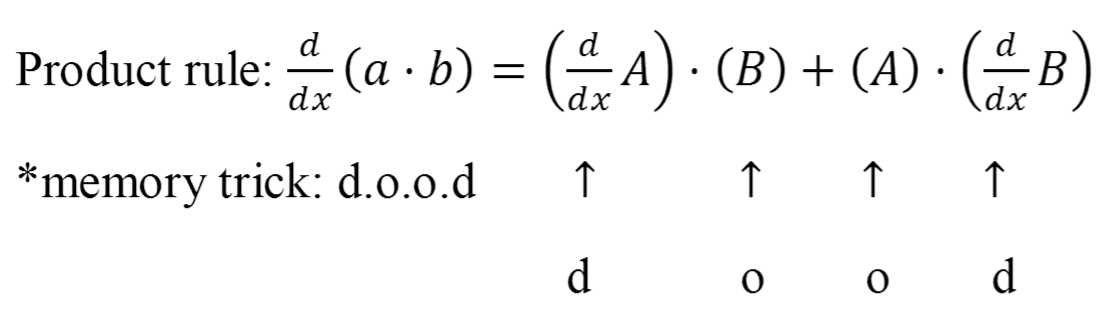# Product rule

### Product rule

In this section, we will learn how to differentiate functions that result from the product of at least two distinct functions using the Product Rule. There are many memory tricks out there that help us remember the Product Rule, the song "hi-de-lo, lo-de-hi", for instance. But since we think they are still a bit too long, we will introduce you a much shorter, cleaner, cooler version – "d.o.o.d" (pronounced as "dude").

#### Lessons• 1.
Differentiate:
a) $y = {x^5}\sin x$
b) $y = {\left( {6{x^2} + x - 4} \right)^5}\sin 2x$